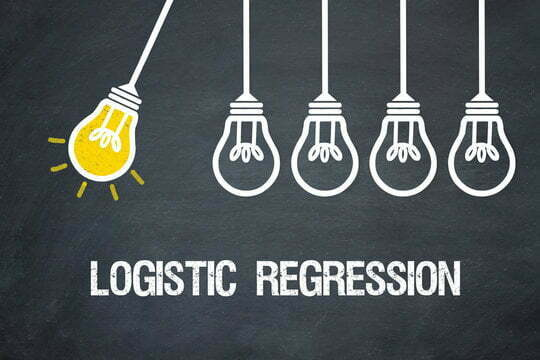# 3 Types of Logistic RegressionStatistical analysis is used in a variety of today’s fields. They give us a way of paring down a variety of variables into easy-to-follow datasets. Some industries that utilize such methods include politics, law enforcement, and food services.

One of the forms of statistical analysis includes logistic regression. We’ll break down three different types of this widely used statistical model.

## 1. Binary Logistic Regression

The first type of logistic regression that we’ll tackle is binary logistic regression. Logistic regression at its core is a statistical model that individuals use to determine the probability of an event happening. Through a logistic regression model, a user will show the relationship between features while then calculating the probability of a certain outcome. This type of statistical analysis includes three separate types of logistic regression. Companies such as data software industry leader TIBCO utilize such logistic regression models. At the head of these different types of logistic regression analysis is binary logistic regression. With binary logistic regression, there are only two possible outcomes for a categorical response.

An example of this would be in the instance of a student passing or failing. As mentioned before, there are only two possible outcomes in this circumstance. There’s not much of an odds ratio in cases of binary logistic regression. The logistic function is set up for only two separate choices. In cases of this binary variable circumstance, the odds of a possible choice can be defined as the probability that a possible outcome is divided up by the other probability that is a non-instance. This form of logistic regression can be used in a variety of circumstances, where the result is either-or. For example, let’s say you’re a bank and you want to predict if a loan applicant will be a bank defaulter or non-defaulter.

You can utilize such predictor variables as loan amounts, monthly installments, employment tenure, delinquency, and debt-to-income ratio to determine how their chances of paying back a loan might look. Using the method of binary logistic regression, you can then decide about the way this applicant might go. Another example includes a doctor trying to decide about the benefits of a new treatment for their patient. They once again utilize this type of logistic regression, combined with other factors (the patient’s blood pressure, hemoglobin level, blood sugar level) to make their decision. Binary logistic regression is one of the most successfully applied forms of logistic regression that people utilize.

## 2. Multinomial Logistic Regression

With multinomial logistic regression, these are circumstances where the response variables usually include three or more variables. These outcome variables will not be in any order, and in some cases have various key differences

Multinomial logistic regression can be used to predict categorical placement as related to category membership. The independent variables that you come across in such circumstances are either dichotomous or continuous. Another possible use for this type of logistic regression includes its use at a restaurant. A restaurant owner can use multinomial logistic regression to figure out if their customers prefer a certain type of food. This might include deciding if a menu needs to be changed to vegan, vegetarian, or meat. Using this type of logistic regression equation is just another way that logistic regression can be used.

## 3. Ordinal Logistic Regression

Our last form of logistic regression is ordinal logistic regression. With this logistic regression equation, there can be three or more variables. You must follow a set of measurements when using the ordinal logistic regression model. With this iteration, for instance, when rating a hotel, you can only use a scale of 1 to 5. For statisticians, this is a simple to use method of logistic regression, due to only being able to use a set scale. Sometimes the methods used in ordinal logistic regression can set the best parameter in determining a straight-line analysis. This can include something along the lines of a 1 to 5 movie rating. Utilizing ordinal logistic regression can help to pare down variables to an easy-to-decipher dataset.